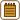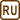## Electric resistance of a constantan and a copper wire

### Task number: 2119

How long is a constantan wire with a diameter of 0.6 mm that has a resistance of 10 Ω? What length of a copper wire of the same diameter would have the same resistance?

• #### Hint

Remember of look up the formula of the resistance of a wire with a uniform cross-section.

• #### Solution

The electrical resistance of a wire with uniform cross-section is given by the formula

$R=\rho\frac{l}{A}.$

We rearrange the formula to express the length of th wire:

$l =\frac{RA}{\rho}.$

We need to calculate the cross-sectional area A of the wire from its diameter d (assuming a cylindrical shape of the wire)

$A=\pi\left(\frac{d}{2}\right)^2.$

Substituting into the previous formula, we get the length of the constantan wire:

$l_{\mathrm{Co}}=\frac{R\pi \left(\frac{d}{2}\right)^2}{\rho_{\mathrm{Co}}}= \frac{R\pi d^2}{4\rho_\mathrm{Co}}.$

where ρCo is the constantat resistivity.

Analogically, we get the length of the copper wire with resistivity ρCu

$l_{\mathrm{Cu}}=\frac{R\pi \left(\frac{d}{2} \right)^2}{\rho_{\mathrm{Cu}}}=\frac{R\pi d^2}{4\rho_\mathrm{Cu}}.$
• #### Notation and numerical calculation

 $$R = 10\,\mathrm\Omega$$ Wire resistance $$d = 0.6\,\mathrm{mm}=0.6{\cdot}10^{-3}\, \mathrm m$$ Wire diameter $$l_{\mathrm{Co}}= ?\,\mathrm{ (m)}$$ Constantan wire length $$l_{\mathrm{Cu}}= ?\,\mathrm{ (m)}$$ Copper wire length From the tables: $$\rho_{\mathrm{Co}}=0.5\,\mathrm{\mu \Omega m}=0.5{\cdot}10^{-6}\,\mathrm{\Omega m}$$ Constantan electrical resistivity $$\rho_{\mathrm{Cu}}=0.018\,\mathrm{\mu \Omega m}=0.018{\cdot}10^{-6}\,\mathrm{\Omega m}$$ Copper electrical resistivity

$l_{\mathrm{Co}}=\frac{R\pi d^2}{4\rho_{Co}}=\frac{10\,\pi \left(0.6{\cdot} 10^{-3}\right)^2}{4{\cdot} 0.5{\cdot} 10^{-6}}\,\mathrm m = 5.7\, \mathrm m$ $l_{\mathrm{Cu}}=\frac{R\pi d^2}{4\rho_{Cu}}=\frac{10\,\pi \left(0.6{\cdot} 10^{-3}\right)^2}{4{\cdot} 0.018{\cdot} 10^{-6}} \,\mathrm m = 157\, \mathrm m$
• #### Odpověď

The length of the constantan wire is 5.7 m. The copper wire would have to be 157 m long.